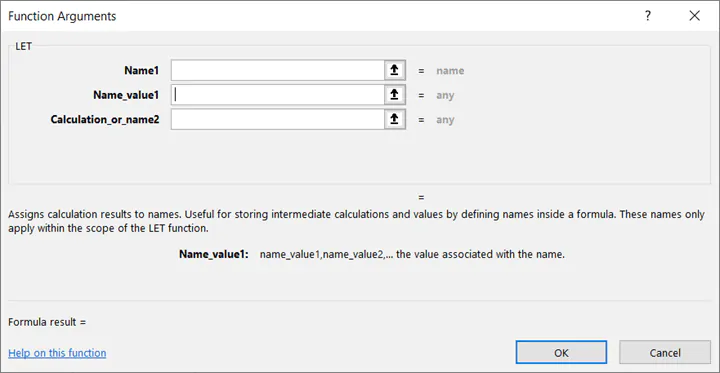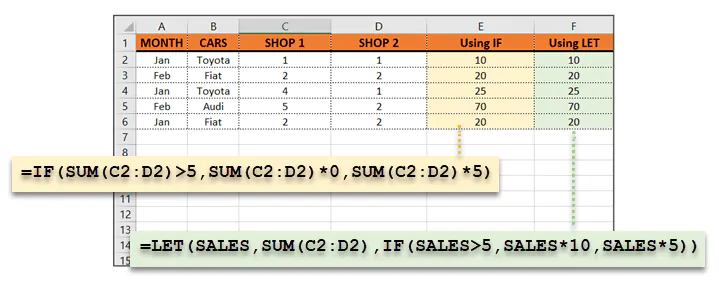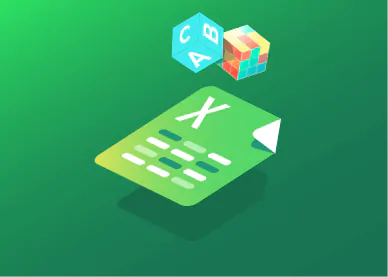# The New Excel LET FunctionSaikat Basu#### Join the Excel conversation on Slack

Ask a question or join the conversation for all things Excel on our Slack channel.

The Excel LET function can help you make complex formulas easier to read and use. With the LET function, you can assign a unique name or identifier to the values in a formula. This name is like a “container” for any value that you can store temporarily and reuse in your calculations as many times as you want.

You assign names once but reuse them multiple times. The value assigned to a name can be constant values or dynamic values that result from a calculation. Excel allows 126 name/value pairs with the LET function.

The LET function works just like variables in any programming language. Even Excel beginners can use this simple function to manage spreadsheet data in several ways:

• Makes complex formulas simpler to manage.
• Makes calculations easier to read and understand.
• Makes it remember complex formulas with descriptive names.
• Makes spreadsheets perform better as Excel calculates a formula once and assigns it to the given name.

## How to get the LET function in Excel

The LET function is available in Excel for Microsoft 365 for Windows and Mac, and Excel for the web. The function was gradually rolled out from July 2020. Do note that the LET function may not be available to you yet if you are not a Microsoft 365 subscriber.

## Syntax of the LET function

Microsoft Excel defines it as a Text function with this syntax:

``LET (Name1, Value1, [Name2…], [Value2…], Calculation)``• Name1: The name for the 1st value
• Value1: The value assigned to the 1st name
• Calculation: The calculation the function performs with the names and values. This is always the final parameter, and it can use any combination of the names defined in the LET function.

For instance, when you use one name-value pair with the LET function:

``=LET(NAME, 100, SUM(NAME, 1))``

Here, we set up NAME as the variable which holds the value of 100. The next part of the formula uses this value and adds “1” with the SUM function. The formula returns “101” as the result.

Here:

``````name1 = NAME

value1 = 100

Calculation = SUM(NAME,1)```
```

When you want to use more than one name value pair with the LET function:

``=LET(X,1,Y,2,X+Y)``

Here:

``````name1 = X

value1 = 1

name2 = Y

value2 = 2

Calculation = X+Y```
```

## Example of a complex LET function

Use this free Excel file to practice along with the tutorial.

The LET function is more useful when you have a more complex formula with multiple nested functions.

In this example, we add the number of cars sold for each make. If the number of cars sold is greater than five, then the sum is multiplied by a factor of ten. If not, it is multiplied by five.The first results column uses a simple IF statement:

``=IF(SUM(C2:D2)>5,SUM(C2:D2)*10,SUM(C2:D2)*5)``

You can see that the same function (SUM) is repeated three times in the calculation. This can be avoided, and the statement can be leaner with a LET function.

``=LET(SALES,SUM(C2:D2),IF(SALES>5,SALES*10,SALES*5))``

Here:

``````Name = SALES

Value = SUM(C2:D2)

Calculation = IF(SALES>5,SALES*10,SALES*5)``````

Both statements lead to the same result but LET helped to simplify the IF calculation.Want to try your hand at a real-world application of this concept? Check out this Excel challenge using the LET function to simplify several options and their possible outcomes.

## Summary

The Excel LET function is a great way to simplify your formulas and make them easier to read and understand. This is especially useful to make complex formulas and calculations easier to manage and remember. Another bonus is that your spreadsheets will run better as Excel calculates a formula once and assigns it to the given name.

The LET function is currently available in Microsoft 365 and Excel Online, so you may not see it if you are using a previous version of Excel.

To learn more Excel essentials, including more new functions like XLOOKUP, the GoSkills Basic and Advanced Excel course can help. Start learning today with a seven-day free trial to all courses on GoSkills.

Ready to become a certified Excel ninja?